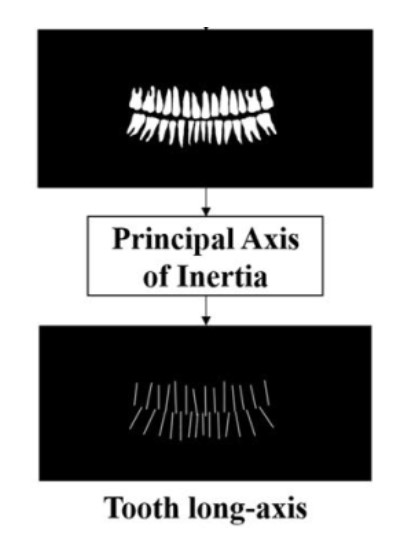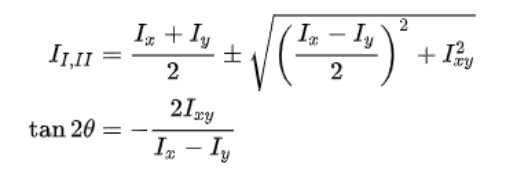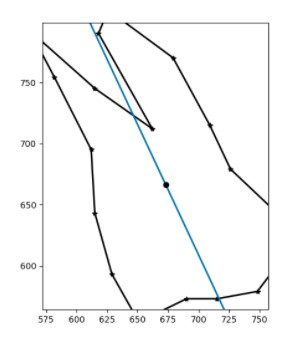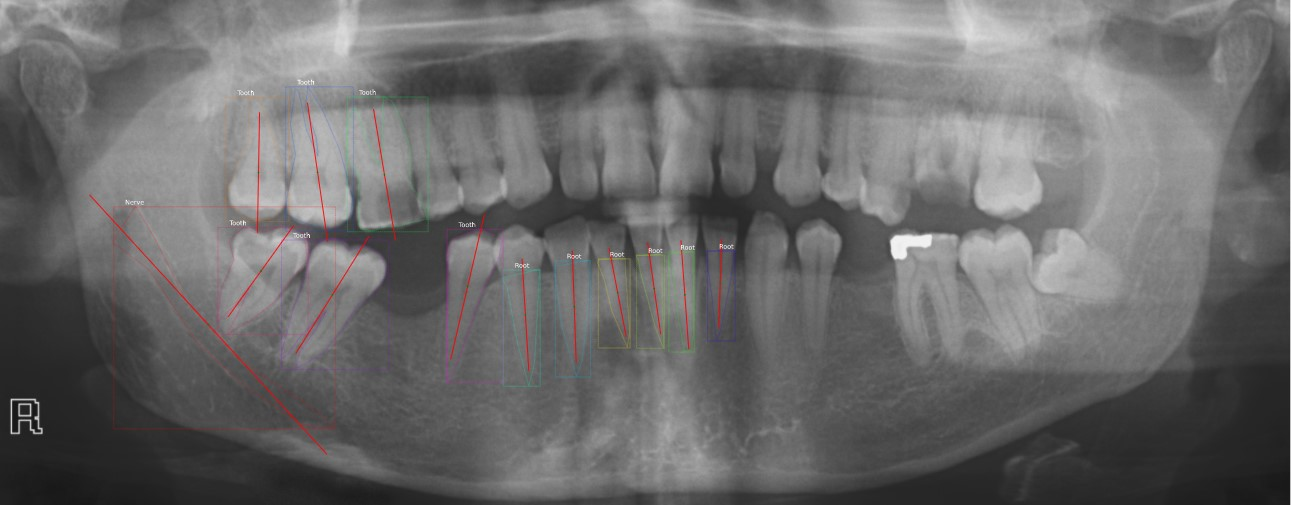# Principal Axis for Tooth

Hi guys, It’s Thurga Shri. I have been working on computing the principal axis for each tooth from the OPG x-ray. In this project, the principal axis is needed to calculate the percentage of bone loss for the classification of staging for periodontitis and also to obtain the angulation for the impacted tooth.Basically, the picture shown above is the desired output of the principal axis which is derived from the mask from the output of Mask-RCNN. Well, to go deeper into how this can be computed, we can look briefly into some formulas and also some codes to look at the built in function to compute the axis (scikit image).

The moments of inertia about principal axes are called principal moments of inertia, and are the maximum and minimum ones, for any angle of rotation of the coordinate system. If Ix, Iy and Ixy are known for the arbitrary centroidal coordinate system x,y, then the principal moments of inertia, I1 and I2, and the orientation of the principal axes, theta, can be found, through :where Ix, Iy and Ixy represent the moments of inertia about the x-axis, moment of inertia about the y-axis, and the product of inertia with respect to x and y axes, respectively

The figure shown below is the principal axis computed for one of the polygons of the tooth using mathematical formula. I have attached the link at the references section.### Compute Principal Axis with Scikit-Image

Thank goodness to the function from scikit image, the principal axis can be computed in just a few lines. The library used is skimage.measure.regionprops; to measure properties of labelled image regions. The link will be attached at the end of this document.

A code snippet to find the principal axis is shown below :

First of all, the image to be fed into this function has to be binary, therefore if it's not already binary, you will have to first convert it to binary image. The figure below is the principal axis computed for every labelled mask, from the centroid (x0, y0) with the green dot extending along the major axis.I hope this information will be useful for you if you were to compute the principal axis for any object. Thank you!

### References:

Link to skimage.measure.regionprops :https://scikit-image.org/docs/dev/auto_examples/segmentation/plot_regionprops.html

Link to computing principal axis with mathematical python script :https://leancrew.com/all-this/2018/01/python-module-for-section-properties/

Link to mathematics of principal axis:http://www.iitg.ac.in/rkbc/me101/Presentation/L19-21.pdf

Synthetic Datasets: Future of Data Collection